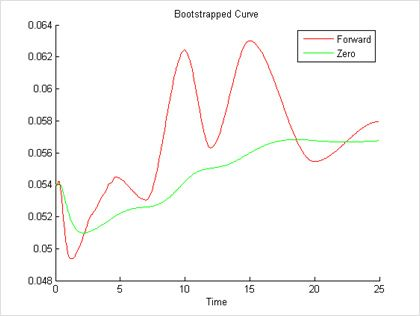Zero Curve

Construct and analyze zero curves

A zero curve is a special type of yield curve that maps interest rates on zero-coupon bonds to different maturities across time. Zero-coupon bonds have a single payment at maturity, so these curves enable you to price arbitrary cash flows, fixed-income instruments, and derivatives. Another type of interest rate curve, the forward curve, is constructed using the forward rates derived from this curve.Bootstrapping an interest rate curve using the zero and forward curves.

Zero curves are separately constructed for government securities and for inter-bank markets.

Zero-coupon bonds are available for a limited number of maturities, so you typically construct zero curves with a combination of bootstrapping and interpolation techniques in order to build a continuous curve. Once you construct these curves, you can then use them to derive other curves such as the forward curve and to price financial instruments.

For more information, see MATLAB® toolboxes for finance, data feeds, financial instruments, statistics, and curve fitting.

See also: swap curve, yield curve, Financial Toolbox, Econometrics Toolbox, Parallel Computing Toolbox, Global Optimization Toolbox, Deep Learning Toolbox, Curve Fitting Toolbox, Datafeed Toolbox, Statistics and Machine Learning Toolbox, Financial Instruments Toolbox

Risk Management with MATLAB

Develop, manage, review, and challenge internal and regulatory models.